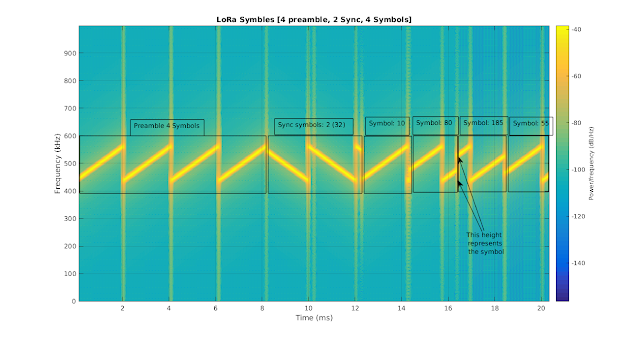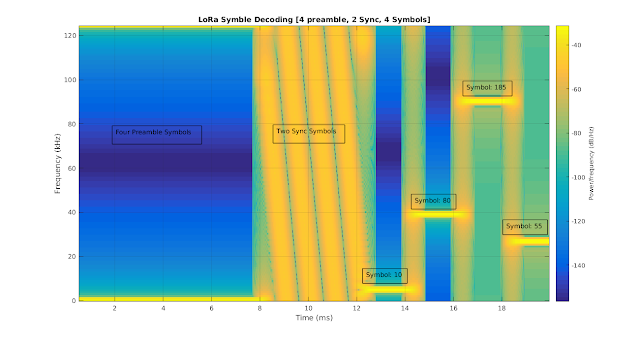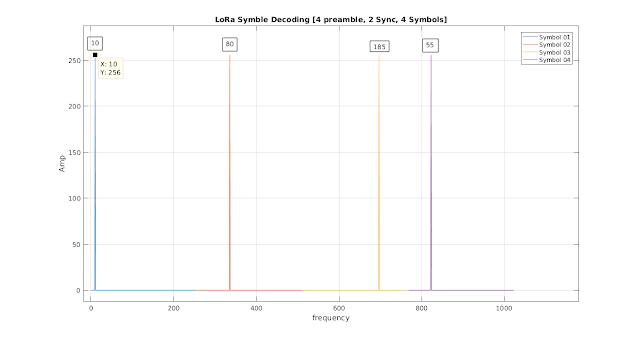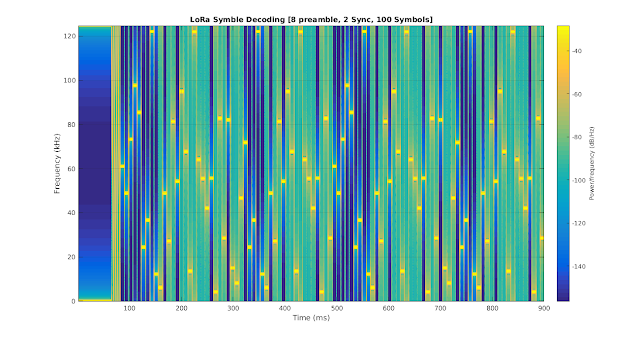### LoRa Decoding

If you are new to LoRa then first go through the following posts:

For matlab codes please refer to : Matlab codes for LoRa Decoding

LoRa Radio Layer message format mainly includes Preamble, Sync message, Payload and CRC.LoRa Radio Layer Format

Preamble: Used to detect the LoRa signals.
Sync Message: Used to detect the starting of the LoRa Payload.
Payload: It contains MAC commands and/or message.
CRC: (only in uplink) used to check block errors.

Concept of Decoding LoRa:
"While encoding LoRa, we cyclic shift the frequency of the LoRa signal. So, if we multiply the received 'frequency shifted LoRa chirp' with 'inverse chirp' we will get the constant frequency signal, with a specific frequency characteristic of the transmitted signal. Now If we take FFT/DFT over the entire symbol then the bin with the highest energy will represent the transmitted symbol."

Let's understand it in detail with an example:

Let's assume we are transmitting a LoRa message which has 4 preamble symbols, 2 synchronisation symbols (32) and 4 message symbols (10,80,185,55). Let's take the spreading factor = 8.

This is how the LoRa message will look like:LoRa symbols
As the spreading factor is 8, so, we can transmit 8 bits over one symbol (0-255). the complete chirp is divided in 256 (as SF = 8) steps and starting frequency represents the symbols.
For example:
For symbol '0' or '256' the message symbol will look like preamble symbol and for '128' the starting frequency will be exactly in the middle.

Now at receiver side this message is multiplied by inverse chirp.

This is how the spectrogram will look like:LoRa Decoded Symbols
After simply multiplying with the inverse chirp you can clearly observe your preamble, sync symbol and transmitted message symbols. Received message symbols has constant frequencies. The frequency (y-axis) represents the symbol and length of the message symbol (x-axis) represents the time-in-the air.

Now after multiplying the received symbol with inverse chirp we take FFT/DFT to check which frequency bin has highest energy.

This is how the symbols will look like:LoRa Decoding FFT
In the above graph the frequencies having highest energy in a symbol (FFT size 256) represents the data. This way the data can be extracted easily.

This is how a spactrogram looks like when you transmit 100 message symbols:LoRa message decoding

Checkout the Matlab codes for LoRa decoding and complete simulation

Have any doubt? Feel free to comment.

If you are a research student and want to sell your work on my Blog here, please reach me on sakshama.ghosliya@gmail.com

1.Hi，very clear description. Is the signal in the figure extracted from the LoRa modem or not?

1.Hello Ning, The signals in the figures are generated by Matlab simulations, they are not extracted from LoRa modem.

2.I saw that you are doing works regarding lora which is the same with me. So what is your goal? Are you trying to reverse lora?

3.Hello Ning, Yes I am trying to reverse the LoRa. What you have done so far?

2.Hi, why the synchronization symbols are reversed, i.e at the time beginning they start from higher frequency to lower. is that has to do with upchirp or downchirp pulses ?

1.Hi Ahmed, Yes the sync symbols (0x32) are downchirp pulses. They are used for time synchronisation of LoRa symbols as the end of the sync is the start of the message symbols.

2.Hello sakshama.. i want to know why the sync symbol is 0x32 exactly

3.Hi...how many bits of data are there in a symbol?

1.Number of Bits depends on Spreading Factor used. In short SF = Number of bits in one symbol.

4.hi sakshama ghoslya

I am working on reversing LoRa PHY functioning. i have one confusion regarding the spreading.
in my simulations, i am transmitting 2^SF chips instead of one symbol. and this is how LoRa works. But here you are saying that a symbol is SF bits long and chirp length is varied as 2^SF (in my simulations, chirp signal has fix number of samples).

moreover, i couldn't understand when you say that
" For symbol '0' or '256' the message symbol will look like preamble symbol and for '128' the starting frequency will be exactly in the middle"

1.Hello Umber,

Here is line to line answer.

1. In one symbol I'm also transmitting 2^SF chips.
2. Here is one symbol or in 2^SF chips how many you can transmit? max. SF bits.
3. Ofcourse chirp length varies on time axis as you vary SF. Look at the LoRa specification modules.
4. Did you check Matlab code (http://sakshamaghoslya.blogspot.in/p/matlab-codes-for-lora-decoding.html), no. of samples are fix here too.

5. Let's say the SF = 8. You can transmit total 8 no. of bits. that means you can transmit from 00000000 (0) to 11111111 (255). If the transmitted information is 0 (00000000) then chirp will start from 0, like a preamble symbol. If the transmitted information is 128 then the modulated chirp will start exactly from the middle. This is how you modulate the chirp. How do you put information over chirps?

5.what i am doing is:
after channel coding, whitening and interleaving
i generates hadamard code of length 2^SF, then perform spread spectrum e.g. if bit is "1" then +code and if bit is "-1" then i replace it with -code.

that was spreading, after it i generate fix length of chirp signal and modulate it with the resultant spreaded sequence.

1.i would suggest you to read about Chirp modulation and symbol generation. You can go through the semtech documents or LoRa US patent.

6.Hello Sir,

Thanks for very clear explanation. Could you please explain how to multiply received chirp with the inverse chirp? How to get constant frequency symbols in figure 3? A example would be really helpful.

Cheers

1.Hello Jiang,

I have given Matlab codes to generate and decode LoRa symbols. Here is the link: http://sakshamaghoslya.blogspot.in/p/matlab-codes-for-lora-decoding.html
(We simple multiply the received symbols to inverse chirp and then take FFT)

2.7.Hi Sakshama,

Nicely written, crystal clear. By the way, I am working on performance analysis of LoRaWAN. I want to ask about LoRa header (explicit mode). Do you know how to obtain the number of symbols used in the header? I need to find out Theader.

Thanks

8.How to detect my garage gate remote control key (433 MHz)? I want to use LoRa SX1278 (433MHz).

9.... I want to use LoRa SX1278 (433MHz) and Arduino (-:

10.11.What are all the input/ data type required for LoRa performance analysis?.kind ki give some suggestions.

12.Hello, I would like to simulate the effects of a bad time synchronization between the real symbol time and the symbol time perceived by the receiver. How can I simulate it with Matlab?